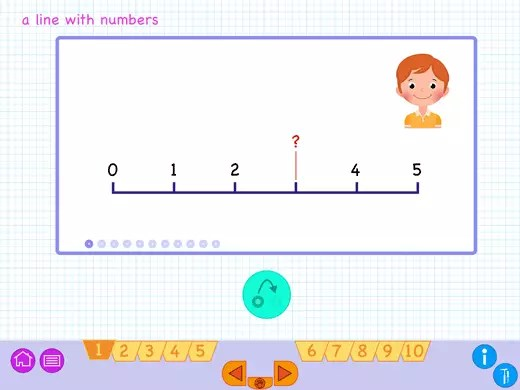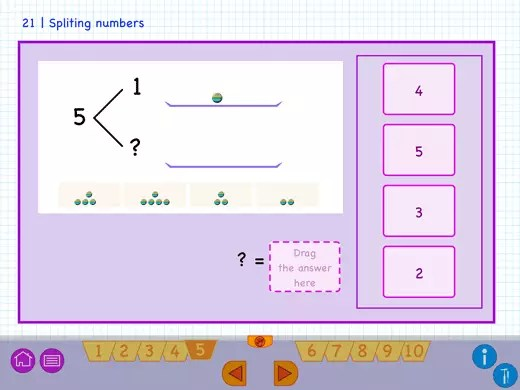Select Page## Plus and Minus app## Learn the fundamental of addition and subtraction with numbers.

The app includes excellent instructions alternated with 59 interactive exercises to practice addition and subtraction with numbers up to 20.
Our “Plus & Minus”-app is ideal to support any maths method, in which you start adding and subtracting numbers.

Topics which are covered in this app, are:

• The line with numbers.
• Addition and subtraction to the number 5.
• Addition and subtraction with 5, 10, 15 and 20.
• Addition and subtraction to the number 20.
• To split numbers.
• Calculating with a bus and train.
• Adding and subtracting with dozens.### The line with numbers

A number line is a line that displays all the integers in order from low to high. First, you practise with up to 5 then finally up to 20. To understand the concept of the number line, you first get short exercises, where you have to add each time the correct number omitted on the line.### To split numbers

You learn to divide numbers with short exercises. You have to break the number into two other values, which together forms the first number. In this way, you will learn the concept of adding and subtracting of numbers.### Splitting by using a train as an example

As soon as you understand the concept of dividing numbers, we will count with numbers above five. You will work with a train as an example, where you have to place cubes on the wagon. In this way, you will learn that 4 + 2 is actually 5 + 1.### Practice addition and subtraction with passengers at the bus stop

After the train, you get the sums with buses. For example: ‘ A bus drives away with twenty passengers. One passenger leaves the bus at the next stop. How many passengers are on the bus? ‘